# Fourier transform of rectangular pulses

• I
PainterGuy
TL;DR Summary
Using Laplace transform to work out the FT of two rectangular pulses. Wanted to know if my work is correct. I did a Matlab code but it didn't produce expected result so wanted to make sure if I worked it out correctly.
Hi,

I was trying to find Fourier transform of two rectangular pulses as shown below. The inverted rectangular pulse has unit height, -1, and lasts from -π to 0. The other rectangular pulse has unit height, 1, and lasts from 0 to π.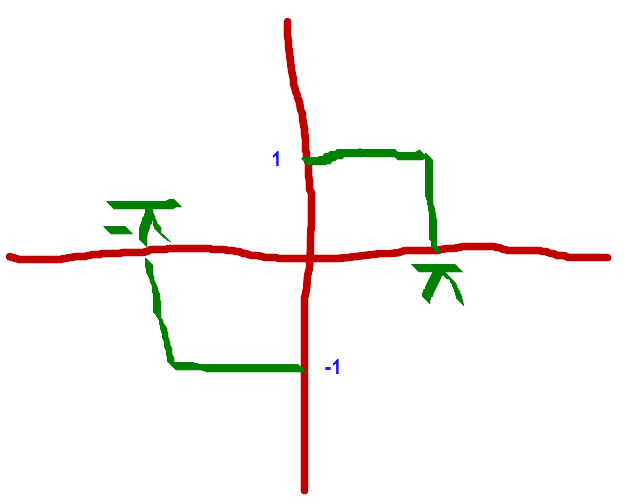I was making use of Laplace transform and its time shifting property to work out the Fourier transform and would like to stick with this method.

Do you think my work is correct? Thank you.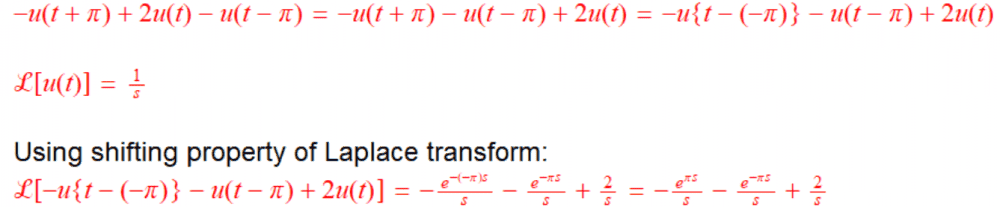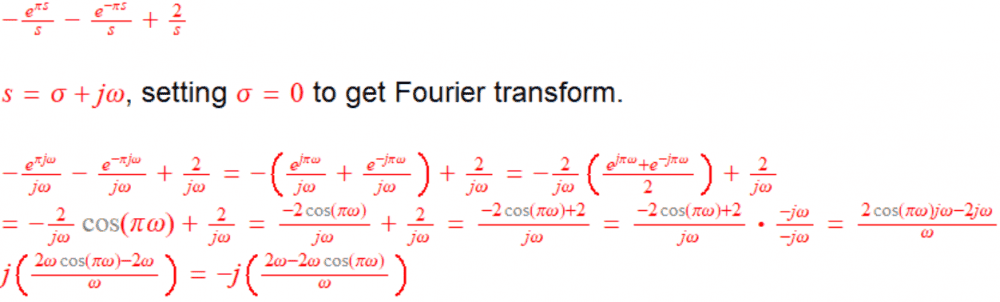Gold Member
Your final answer should be correct if you did the algebra correctly (which I think you did).

Here is why it worked. If you have some function ##f(t)## with Laplace transform ##F(s)## with some region of convergence, and Fourier transform ##\hat{F}(\omega)##, then ##\hat{F}(\omega) = F(j\omega)## only if the region of convergence for ##F(s)## includes ##\sigma = Real(s) = 0##. The region of convergence for your Laplace transform does include ##\sigma=0## so it satisfies that requirement.

However, if your function was just ##u(t)## then the Laplace transform is ##1/s## with region of convergence ##real(s)>0##, so the Fourier transform is not ##\frac{1}{j\omega}##. Instead, the Fourier transform is ##\frac{1}{j\omega} + \pi \delta(\omega)##. Whenever the region of convergence for ##F(s)## is ##real(s)>0## you should expect the Fourier transform to have things like delta functions in it. If the region of convergence for ##F(s)## is ##real(s)>a>0##, then the Fourier transform does not exist at all; one example is ##u(t) e^t## which has Laplace transform ##\frac{1}{s-1}## with region of convergence ##real(s)>1## .

jason

•PainterGuy
PainterGuy
Here is why it worked. If you have some function ##f(t)## with Laplace transform ##F(s)## with some region of convergence, and Fourier transform ##\hat{F}(\omega)##, then ##\hat{F}(\omega) = F(j\omega)## only if the region of convergence for ##F(s)## includes ##\sigma = Real(s) = 0##.

Thank you for your note on the conversion between Laplace transform and Fourier transform. I do have a question about second para but will ask it later.

I checked my work again and it looked okay.

Anyway, I tried to verify it further using a Matlab code. I used trigonometric form of Fourier transform to plot the result. Please see the note below about the trigonometric form.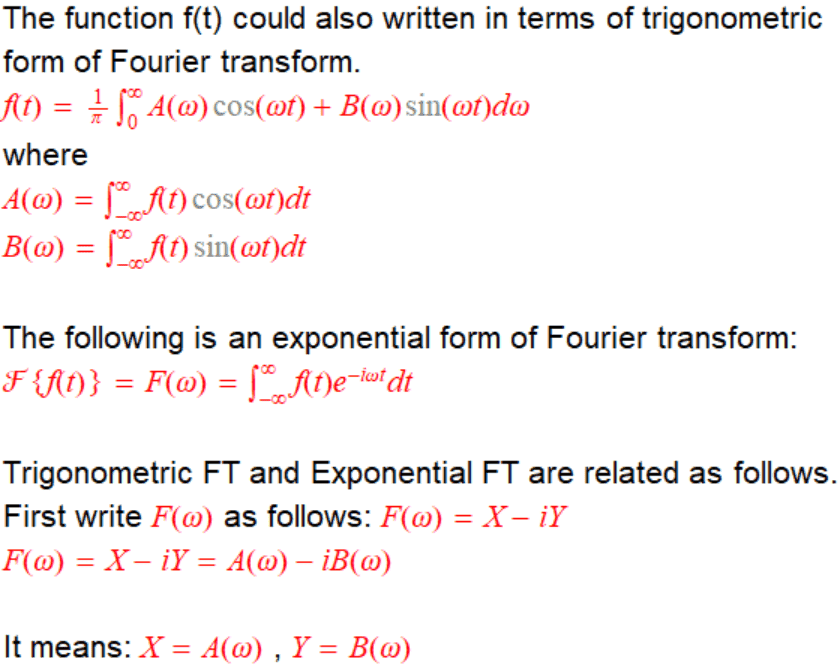I set B(ω) = j{2ω-2ω*cos(πω)}/ω

The following is Matlab code I used. Please note that if you try to run the code, it would produce different warnings but it would work fine.

Matlab:
clear all; close all; clc;

t=linspace(-10,10,12000);

for it=1:12000

f=@(w)(1/pi).*((2.*w-2.*w.*cos(pi.*w))./w).*sin(w.*t(it));
F(it)=integral(f,0,1000);

end

figure('Name','inverse Fourier transform');
plot(t,F,'red');
ylim([-5 5]); % to restrict y axis
hold on;

The plot below was produced using the code above. Note that black dashed lines were drawn by me for clarity. You can see my result is quite weird. I don't think there is a problem with my code but still I cannot figure the problem. In the code I have restricted the y-axis limits. The plot at the bottom, Plot #2, was produced without restricting y-axis.

Plot #1: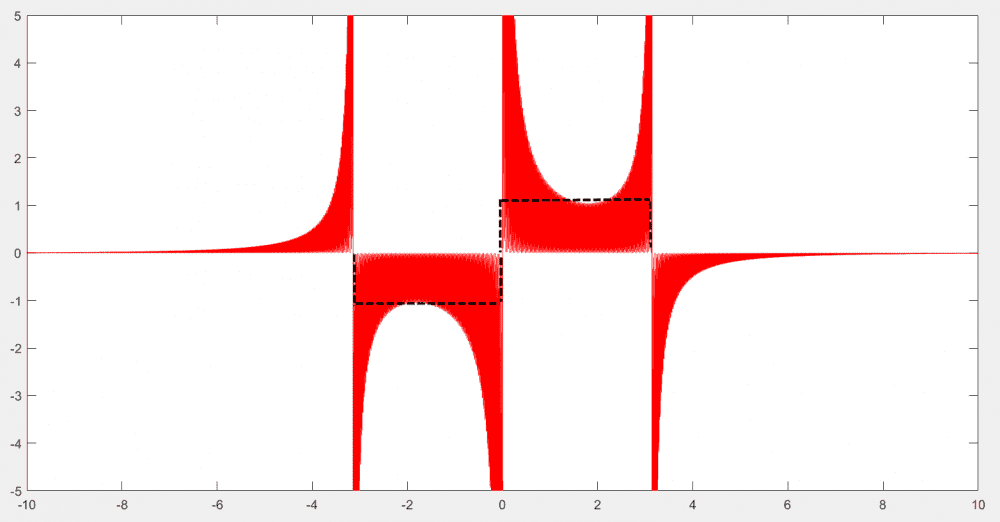Plot #2: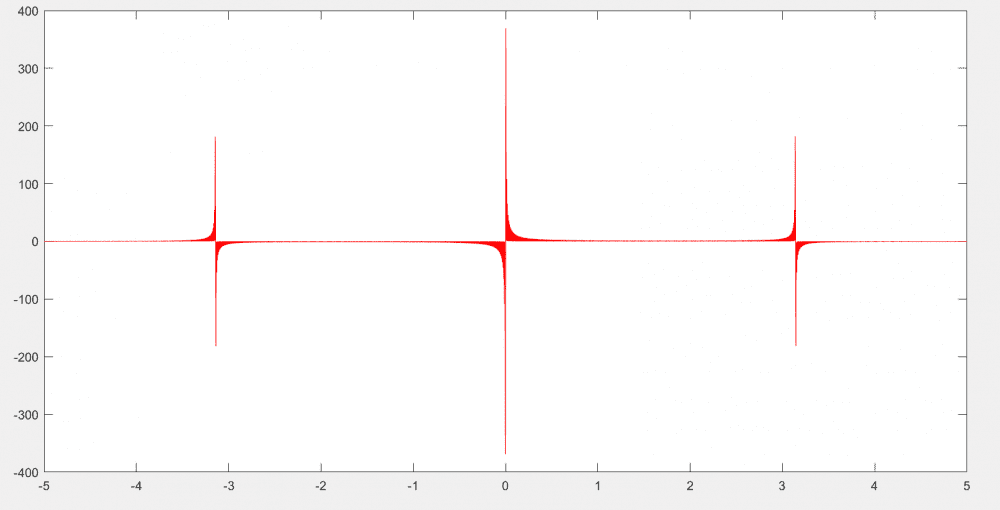Last edited:
Gold Member
I see an error in your algebra in the original post. I happens when you multiply the numerator and denominator both by ##-j \omega##. Also, if you have access to the symbolic toolbox you can use Matlab to do the analytical Fourier transform. The command is Fourier - on the command line type
>> help fourier

to see examples of how to use it. Likewise ifourier is the inverse Fourier transform. Also, the symbolic step function is called heaviside in Matlab.

•PainterGuy
Staff Emeritus
Homework Helper
I noticed you obtained the result
$$F(\omega) = j\frac{2\omega\cos \pi\omega - 2\omega}{\omega},$$ and you used that form in your second post. Is there some reason you didn't cancel the common factor of ##\omega##?

I suggest you calculate the Fourier transform of this function directly so you know what you're shooting for using your method. Then you don't have to guess if you ended up with the right result.

•hutchphd
PainterGuy
I see an error in your algebra in the original post. I happens when you multiply the numerator and denominator both by ##-j \omega##.

Thank you for pointing this out. I have fixed the error below.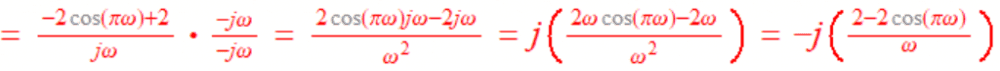It worked as well. The following plot was obtained after fixing the error in previous code.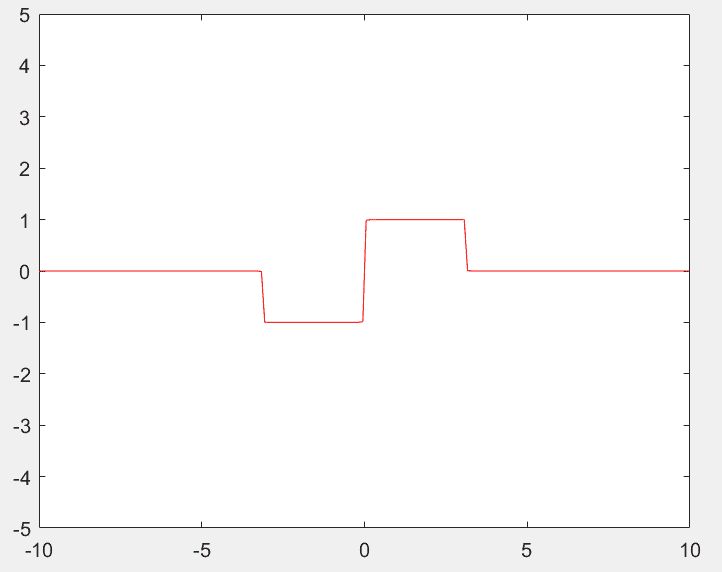I suggest you calculate the Fourier transform of this function directly so you know what you're shooting for using your method.

Thank you for the suggestion. The method is good and I only made an algebraic error, and such an error could occur in the direct Fourier transform as well.